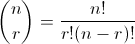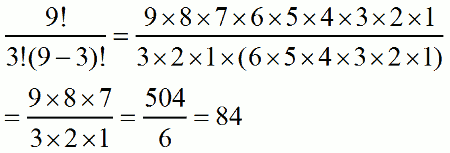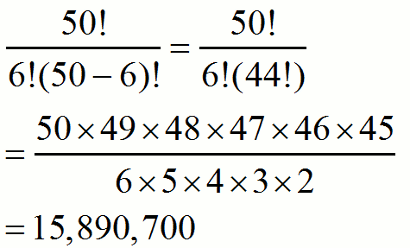# Combinations

If 3 players are selected from a team of 9, how many different combinations are possible? First, we need to define what a combination means. In mathematical terms, a combination is an subset of items from a larger set such that the order of the items does not matter. For example, if John, Fred, and Bill are selected from the team, that is considered the same combination as Fred, John, and Bill.

Now, how do we calculate the number of possible combinations? Use the following formula:First, let me explain the notation on the left. That means that from a group of n objects, we are selecting r of them. It's just a standard notation used for combinations, but you might also see something like nCr used instead.

The rest of the formula is more straightforward. Remember that the ! means factorial, the product of that number and all positive integers less than it. For example, 4! = 4*3*2*1.

In the original example I gave, we wanted the number of combinations when selecting 3 out of 9:Notice how a good portion of the multiplication cancelled out. There's no need to calculation 9! all the way out when the 6! below it will just cancel out everything except 9*8*7. In the end, we see that there are 84 ways to pick 3 people from a group of 9 as long as order does not matter.

Consider another example. If a traveller has the option of visiting any 6 of the 50 United States, but doesn't care in which order he sees them, how many different trips are possible? Ignore, of course, the fact that many of these combinations would be very random and unnecessarily complicated!

The problem is a simple combination. There are 50 total states, and we must select 6:There are an astonishing 15 million different groups of 6 states!

Hopefully this gets you started with combinations. For more help, consider these other lessons on combinations, or this page that explains the difference between a combination and a permutation.